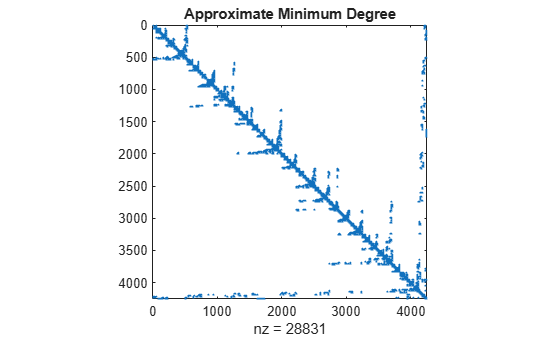# Graphical Representation of Sparse Matrices

This example shows the finite element mesh for a NASA airfoil, including two trailing flaps. More information about the history of airfoils is available at NACA Airfoils (nasa.gov).

The data is stored in the file `airfoil.mat`. The data consists of 4253 pairs of (x,y) coordinates of the mesh points. It also contains an array of 12,289 pairs of indices, (i,j), specifying connections between the mesh points.

Load the data file into the workspace.

`load airfoil`

### View Finite Element Mesh

First, scale `x` and `y` by ${2}^{-32}$ to bring them into the range $\left[0,1\right]$. Then form a sparse adjacency matrix from the (i,j) connections and make it positive definite. Finally, plot the adjacency matrix using (x,y) as the coordinates for the vertices (mesh points).

```% Scaling x and y x = pow2(x,-32); y = pow2(y,-32); % Forming the sparse adjacency matrix and making it positive definite n = max(max(i),max(j)); A = sparse(i,j,-1,n,n); A = A + A'; d = abs(sum(A)) + 1; A = A + diag(sparse(d)); % Plotting the finite element mesh gplot(A,[x y]) title('Airfoil Cross-Section')```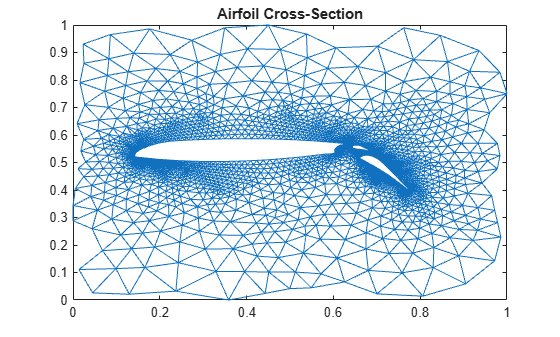### Visualize Sparsity Pattern

You can use `spy` to visualize the nonzero elements in a matrix, so it is a particularly useful function to see the sparsity pattern in sparse matrices. `spy(A)` plots the sparsity pattern of the matrix `A`.

```spy(A) title('Airfoil Adjacency Matrix')```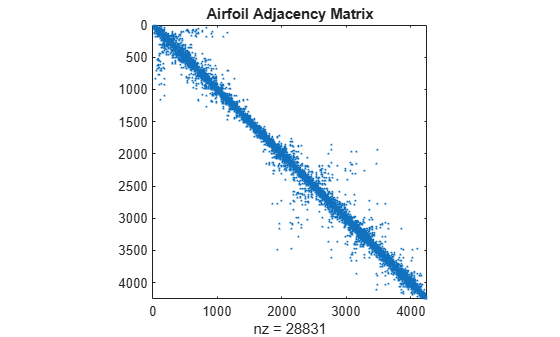### Symmetric Reordering - Reverse Cuthill-McKee

`symrcm` uses the Reverse Cuthill-McKee technique for reordering the adjacency matrix. `r = symrcm(A)` returns a permutation vector `r` such that `A(r,r)` tends to have its diagonal elements closer to the diagonal than `A`. This form is a good preordering for LU or Cholesky factorization of matrices that come from "long, skinny" problems. It works for both symmetric and nonsymmetric matrices.

```r = symrcm(A); spy(A(r,r)) title('Reverse Cuthill-McKee')```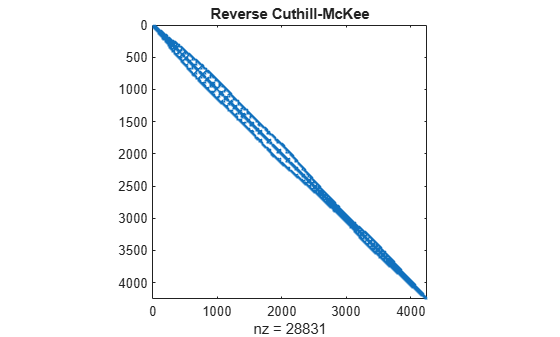### Symmetric Reordering - Column Permutations

Use `j = COLPERM(A)` to return a permutation vector that reorders the columns of the sparse matrix `A` in nondecreasing order of nonzero count. This form is sometimes useful as a preordering for LU factorization, as in `lu(A(:,j))`.

```j = colperm(A); spy(A(j,j)) title('Column Count Reordering')```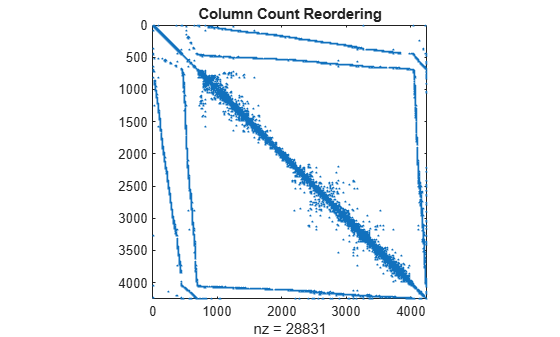### Symmetric Reordering - Symmetric Approximate Minimum Degree

`symamd` gives a symmetric approximate minimum degree permutation. For a symmetric positive definite matrix `A`, the command `p = symamd(S)` returns the permutation vector `p` such that `S(p,p)` tends to have a sparser Cholesky factor than `S`. Sometimes `symamd` works well for symmetric indefinite matrices too.

```m = symamd(A); spy(A(m,m)) title('Approximate Minimum Degree')```